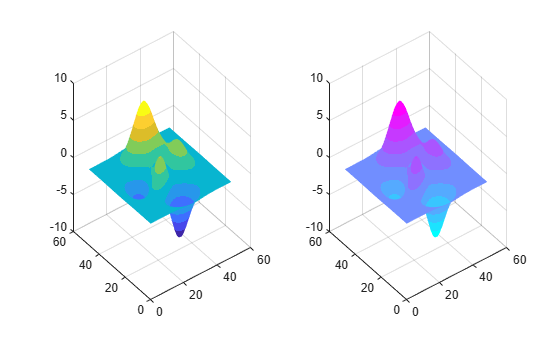# Change Color Scheme Using a Colormap

MATLAB® uses a default color scheme when it displays visualizations such as surface plots. You can change the color scheme by specifying a colormap. Colormaps are three-column arrays containing RGB triplets in which each row defines a distinct color.

For example, here is a surface plot with the default color scheme.

```f = figure; surf(peaks);```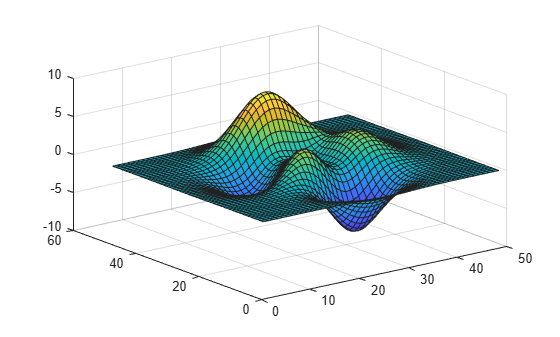The following command changes the colormap of the current figure to `winter`, one of several predefined colormaps (see Colormaps for a full list).

`colormap winter;`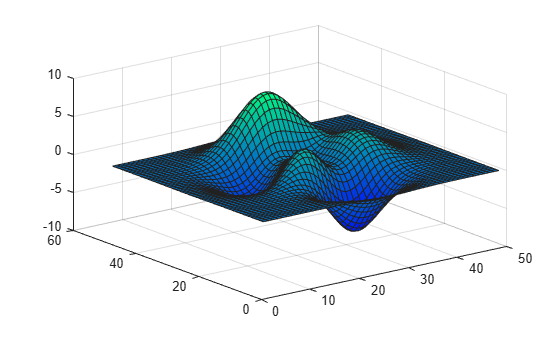If you have multiple figures open, pass the `Figure` object as the first argument to the `colormap` function.

`colormap(f,hot);`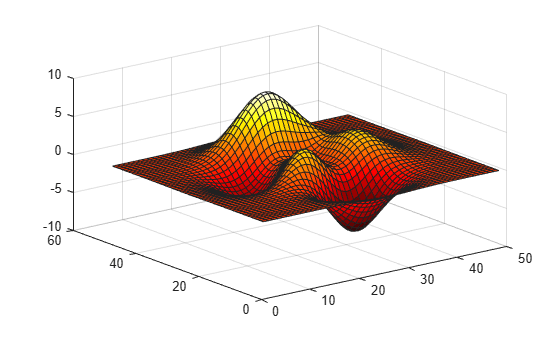Each predefined colormap provides a palette of 256 colors by default. However, you can specify any number of colors by passing a whole number to the predefined colormap function. For example, here is the `hot` colormap with ten entries.

```c = hot(10); colormap(c);```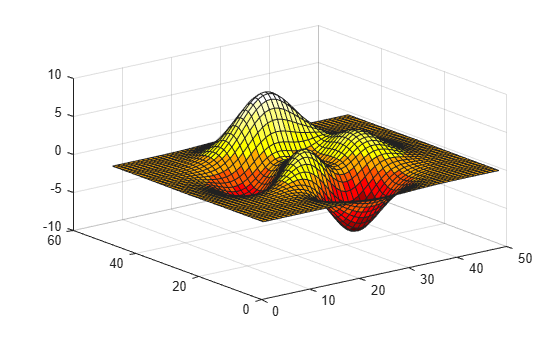You can also create your own colormap as an m-by-3 array. Each row in the array contains the red, green, and blue intensities of a different color. The intensities are in the range [0,1]. Here is a simple colormap that contains three entries.

```mycolors = [1 0 0; 1 1 0; 0 0 1]; colormap(mycolors);```If you are working with multiple axes, you can assign a different colormap to each axes by passing the axes object to the `colormap` function.

```tiledlayout(1,2) ax1 = nexttile; surf(peaks); shading interp; colormap(ax1,parula(10)); ax2 = nexttile; surf(peaks); shading interp; colormap(ax2,cool(10));```